• ## 欧氏距离

万次阅读 2016-03-03 12:49:06
欧氏距离定义： 欧氏距离（ Euclidean distance）是一个通常采用的距离定义，它是在m维空间中两个点之间的真实距离。 在二维和三维空间中的欧式距离的就是两点之间的距离，二维的公式是 d = sqrt((x1-x2)^+(y1-y2)...
欧氏距离定义： 欧氏距离（ Euclidean distance）是一个通常采用的距离定义，它是在m维空间中两个点之间的真实距离。

在二维和三维空间中的欧式距离的就是两点之间的距离，二维的公式是
d = sqrt((x1-x2)^+(y1-y2)^)
三维的公式是
d=sqrt(x1-x2)^+(y1-y2)^+(z1-z2)^)
推广到n维空间，欧式距离的公式是
d=sqrt( ∑(xi1-xi2)^ ) 这里i=1,2..n
xi1表示第一个点的第i维坐标,xi2表示第二个点的第i维坐标
n维欧氏空间是一个点集,它的每个点可以表示为(x(1),x(2),...x(n)),其中x(i)(i=1,2...n)是实数,称为x的第i个坐标,两个点x和y=(y(1),y(2)...y(n))之间的距离d(x,y)定义为上面的公式.
欧氏距离看作信号的相似程度。 距离越近就越相似，就越容易相互干扰，误码率就越高。
目前该距离也会用于Web2.0的数据相似程度的分析，例如：用户喜好的相似程度。
那么为什么是两变量的差值平方和呢？
想想勾股定律吧， a2+b2=c2。
PS: 可用于距离统计量的还有马氏距离，两项距离，明氏距离，另外还包括相关系数的调整及夹角余弦等相关知识。

展开全文• 欧氏距离(Euclidean Distance) 欧氏距离是最容易直观理解的距离度量方法，我们小学、初中和高中接触到的两个点在空间中的距离一般都是指欧氏距离。 Python 实现计算欧氏距离 Python import ...


欧氏距离(Euclidean Distance)
欧氏距离是最容易直观理解的距离度量方法，我们小学、初中和高中接触到的两个点在空间中的距离一般都是指欧氏距离。Python 实现计算欧氏距离

Python

import numpy as np
x=np.random.random(10)
y=np.random.random(10)

#方法一：根据公式求解
d1=np.sqrt(np.sum(np.square(x-y)))

#方法二：根据scipy库求解
from scipy.spatial.distance import pdist
X=np.vstack([x,y])
d2=pdist(X)

123456789101112

import numpy as npx=np.random.random(10)y=np.random.random(10) #方法一：根据公式求解d1=np.sqrt(np.sum(np.square(x-y))) #方法二：根据scipy库求解from scipy.spatial.distance import pdistX=np.vstack([x,y])d2=pdist(X)zeropython 微信公众号
5868037 QQ号
5868037@qq.com QQ邮箱


展开全文• 'z=mydist(w,p)' 计算两个向量 w:SxR 和 p:RxQ 之间的欧氏距离，并返回 z:SxQ，w 的行和 p 的列之间的距离。 此函数与 Matlab 'dist(w,p)' 的作用相同，但计算所需的内存要少得多。 它可以帮助克服神经网络中较大...matlab
• python 欧氏距离距离Prerequisite: 先决条件： Defining a Vector using list 使用列表定义向量 Defining Vector using Numpy 使用Numpy定义向量 In mathematics, the Euclidean distance is an ordinary ...
python 欧氏距离距离Prerequisite:
先决条件：
Defining a Vector using list 使用列表定义向量 Defining Vector using Numpy 使用Numpy定义向量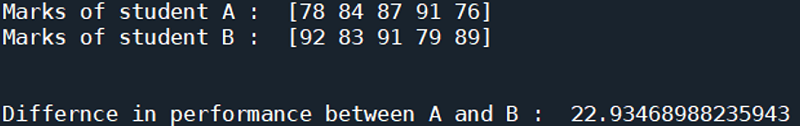In mathematics, the Euclidean distance is an ordinary straight-line distance between two points in Euclidean space or general n-dimensional space. If two students are having their marks of all five subjects represented in a vector (different vector for each student), we can use the Euclidean Distance to quantify the difference between the students' performance.
在数学中， 欧几里德距离是欧几里德空间或一般n维空间中两点之间的一条普通直线距离。 如果两个学生在一个向量(每个学生的不同向量)中表示了所有五个科目的成绩，则我们可以使用欧几里得距离来量化学生表现之间的差异。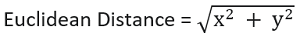x, y are the vectors in representing marks of student A and student B respectively.
x ， y分别是表示学生A和学生B的分数的向量。
欧几里得距离示例的Python代码 (Python code for Euclidean distance example)
# Linear Algebra Learning Sequence
# Euclidean Distance Example

import numpy as np

a = np.array([78, 84, 87, 91, 76])
b = np.array([92, 83, 91, 79, 89])

# Finding the euclidean distance
dis = np.linalg.norm(a-b)

print('Marks of student A : ', a)
print('Marks of student B : ', b)

print('\n\nDiffernce in performance between A and B : ', dis)

Output:
输出：
A :  [ 2  4  8  9 -6]
B :  [2 3 1 7 8]

Cosine Similarity between A and B :  0.2440982792118955


翻译自: https://www.includehelp.com/python/euclidean-distance-example.aspxpython 欧氏距离距离
展开全文python 机器学习 numpy 深度学习 人工智能
• 余弦相似度和欧氏距离Photo by Markus Winkler on Unsplash Markus Winkler在Unsplash上拍摄的照片 This is a quick and straight to the point introduction to Euclidean distance and cosine similarity with a ...
余弦相似度和欧氏距离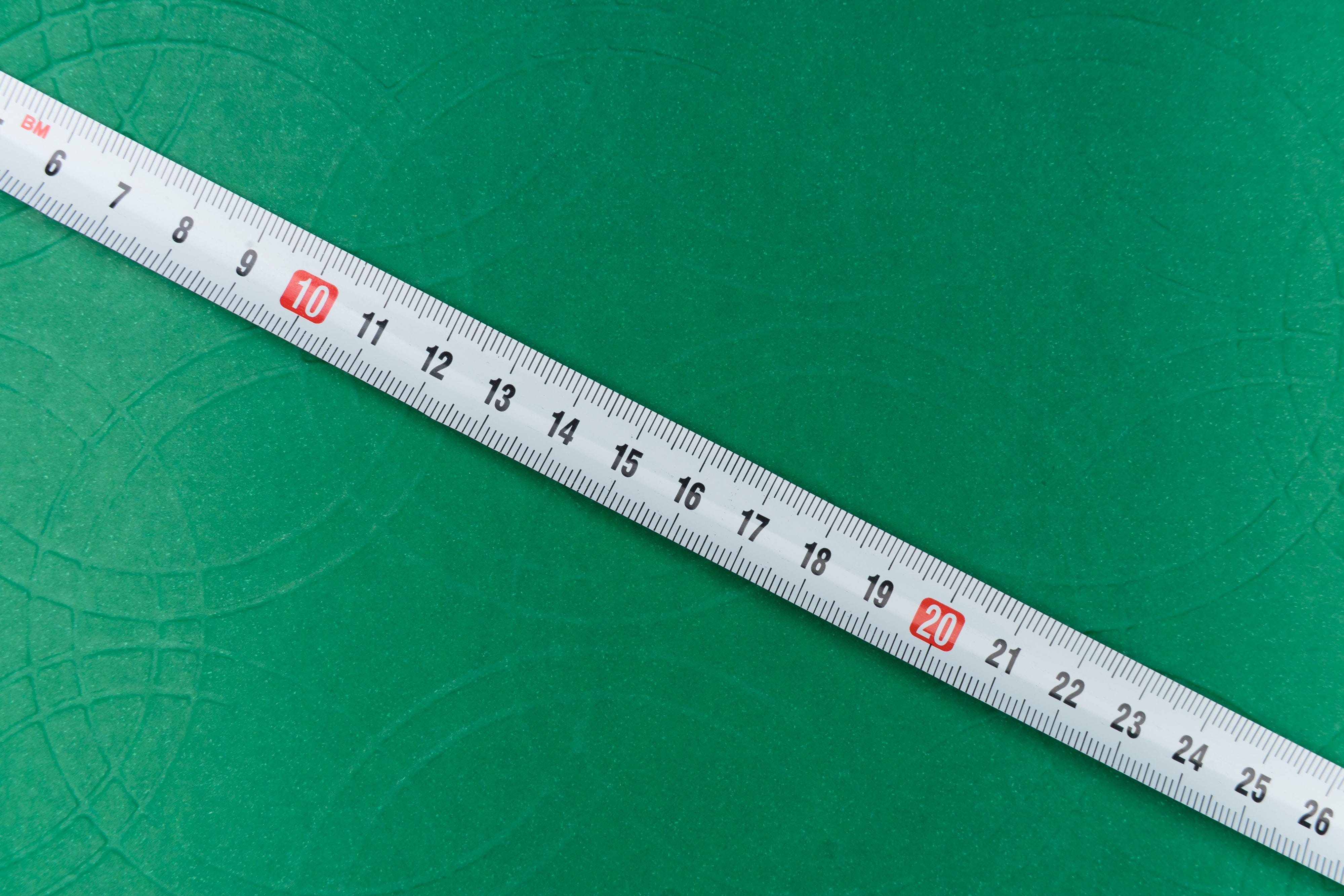Photo by Markus Winkler on UnsplashThis is a quick and straight to the point introduction to Euclidean distance and cosine similarity with a focus on NLP. 这是对欧氏距离和余弦相似度的快速而直接的介绍，重点是NLP。
欧氏距离 (Euclidean Distance)
The Euclidean distance metric allows you to identify how far two points or two vectors are apart from each other. 欧几里德距离度量标准可让您确定两个点或两个向量彼此相距多远。
Now suppose you are a high school student and you have three classes. A math class, a philosophy class, and a psychology class. You want to check the similarity between these classes based on the words your professors use in class. For the sake of simplicity, let’s consider these two words: “theory” and “harmony”. You could then create a table like this to record the occurrence of these words in each class: 现在假设您是一名高中生，您有3个班级。 数学课，哲学课和心理学课。 您想根据您的教授在课堂上使用的单词来检查这些课程之间的相似性。 为了简单起见，让我们考虑以下两个词：“理论”和“和谐”。 然后，您可以创建一个像这样的表来记录每个类中这些单词的出现情况：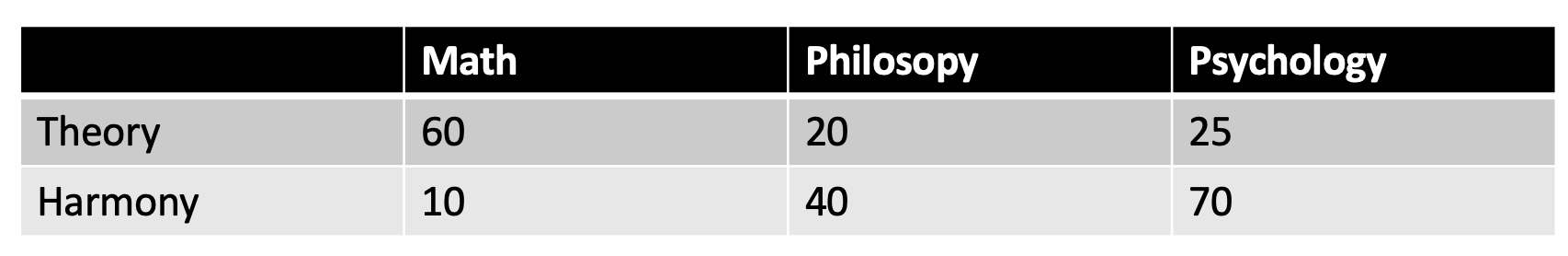In this table, the word “theory” is repeated 60 times in math class, 20 times in philosophy class, and 25 times in psychology class whereas the word harmony is repeated 10, 40, and 70 times in math, philosophy, and psychology classes respectively. Let’s translate this data into a 2D plane. 在此表中，“理论”一词在数学课中重复了60次，在哲学课中重复了20次，在心理学课中重复了25次，而在数学，哲学和心理学课中，“和谐”一词重复了10、40和70次分别。 让我们将此数据转换为2D平面。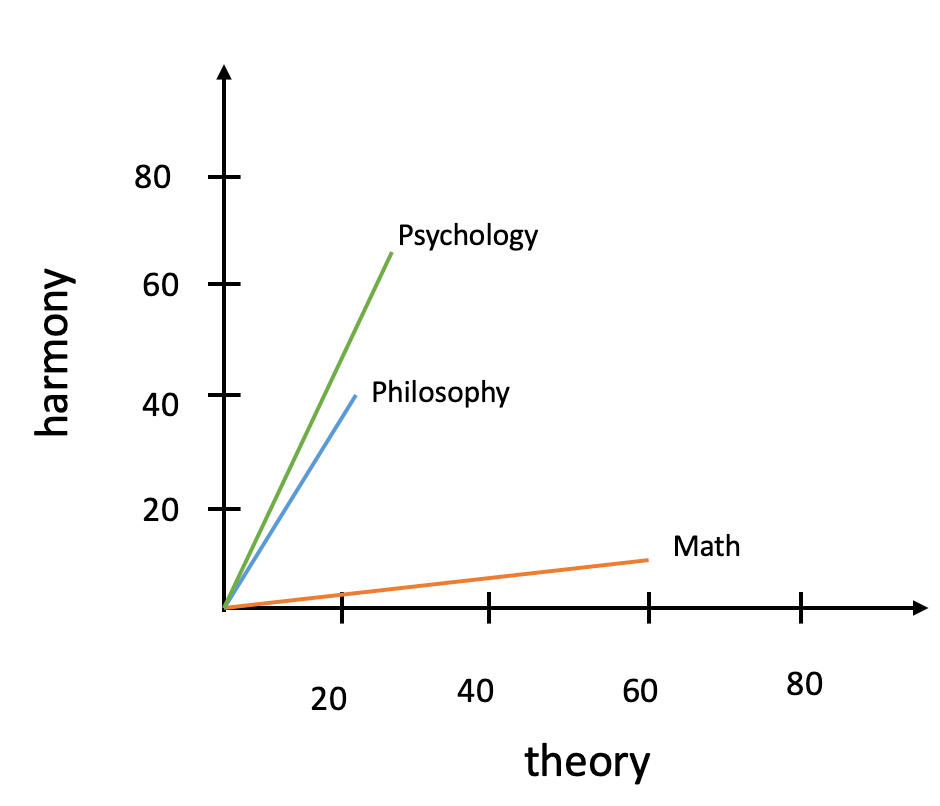The Euclidean distance is simply the distance between the points. In the graph below. 欧几里得距离就是点之间的距离。 在下图中。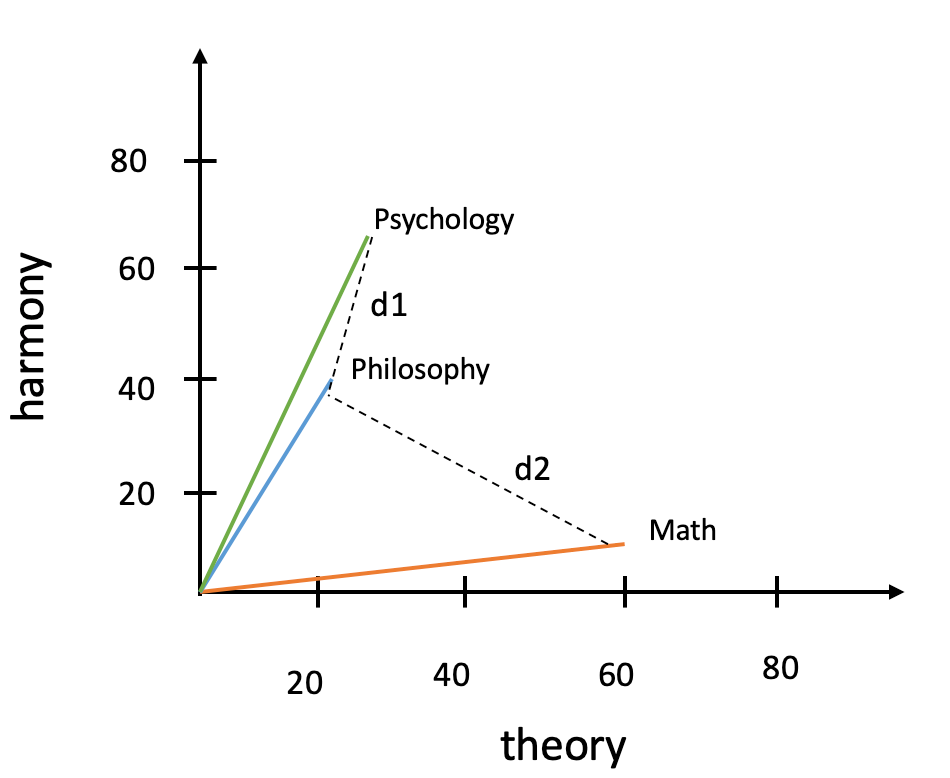You can see clearly that d1 which is the distance between psychology and philosophy is smaller than d2 which is the distance between philosophy and math. But how do you calculate d1 and d2? 您可以清楚地看到，心理学与哲学之间的距离d1小于哲学与数学之间的距离d2。 但是，如何计算d1和d2？
The generic formula is the following. 通用公式如下。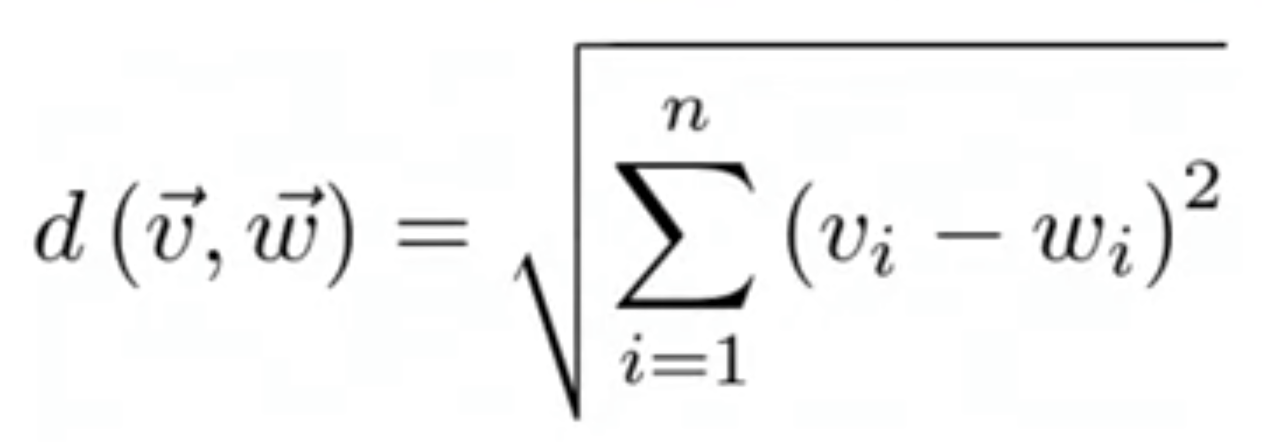In our case, for d1, d(v, w) = d(philosophy, psychology), which is: 在我们的情况下，对于d1， d(v, w) = d(philosophy, psychology) ，即：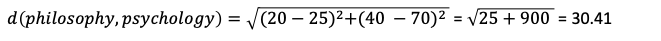And d2 和d2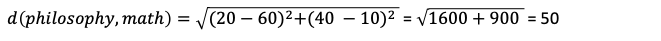As expected d2 > d1. 如预期的那样，d2> d1。
How to do this in python? 如何在python中做到这一点？
import numpy as np# define the vectorsmath = np.array([60, 10])philosophy = np.array([20, 40])psychology = np.array([25, 70])# calculate d1d1 = np.linalg.norm(philosophy - psychology)# calculate d2d2 = np.linalg.norm(philosophy - math) 余弦相似度 (Cosine Similarity)
Suppose you only have 2 hours of psychology class per week and 5 hours of both math class and philosophy class. Because you attend more of these two classes, the occurrence of the words “theory” and “harmony” will be greater than for the psychology class. Thus the updated table: 假设您每周只有2个小时的心理学课，而数学课和哲学课则只有5个小时。 由于您参加这两个课程中的更多课程，因此“理论”和“和谐”一词的出现将比心理学课程中的要大。 因此，更新后的表：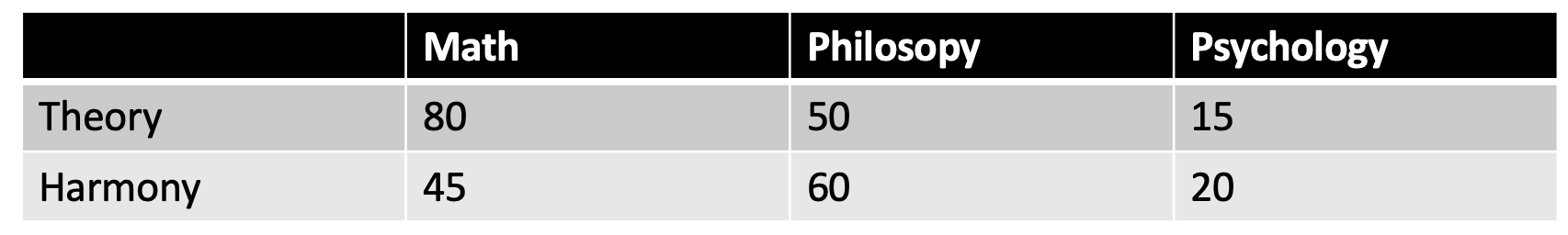And the updated 2D graph: 以及更新后的2D图形：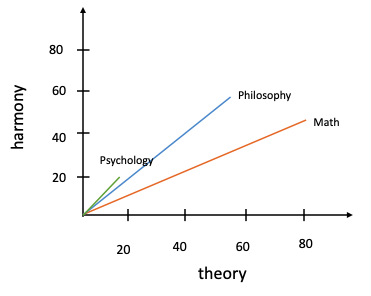Using the formula we’ve given earlier for Euclidean distance, we will find that, in this case, d1 is greater than d2. But we know psychology is closer to philosophy than it is to math. The frequency of the courses, trick the Euclidean distance metric. Cosine similarity is here to solve this problem. 使用我们先前给出的欧几里得距离公式，我们会发现，在这种情况下，d1大于d2。 但是我们知道心理学比数学更接近于哲学。 课程的频率欺骗欧几里德距离度量标准。 余弦相似度在这里解决了这个问题。
Instead of calculating the straight line distance between the points, cosine similarity cares about the angle between the vectors. 余弦相似度关心的是矢量之间的角度，而不是计算点之间的直线距离。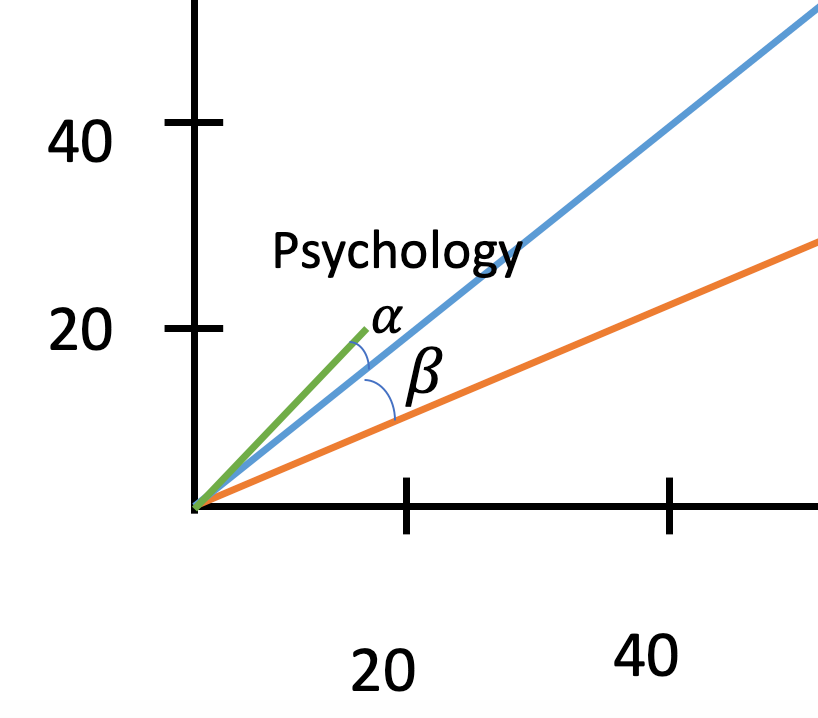Zooming in on the graph, we can see that the angle α, is smaller than the angle β. That’s all cosine similarity wants to know. In other words, the smaller the angle, the closer the vectors are to each other. 放大该图，我们可以看到角度α小于角度β。 这就是所有余弦相似度想要知道的。 换句话说，角度越小，向量彼此越接近。
The generic formula goes as follows 通用公式如下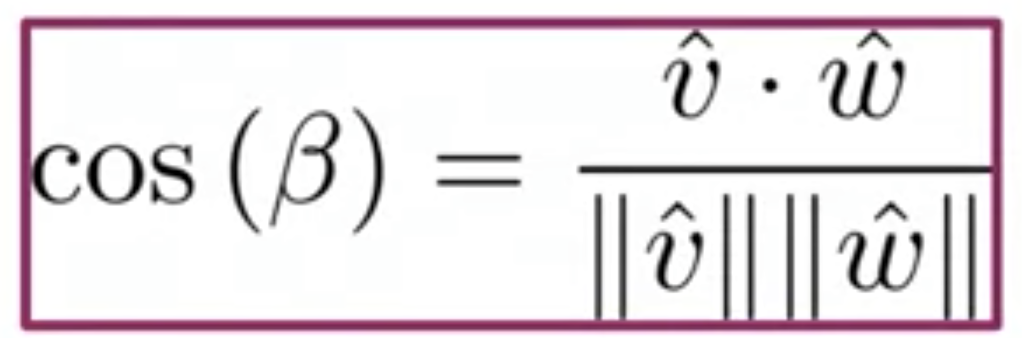β is the angle between the vectors philosophy (represented by v) and math (represented by w). β是向量原理(用v表示)和数学(用w表示)之间的夹角。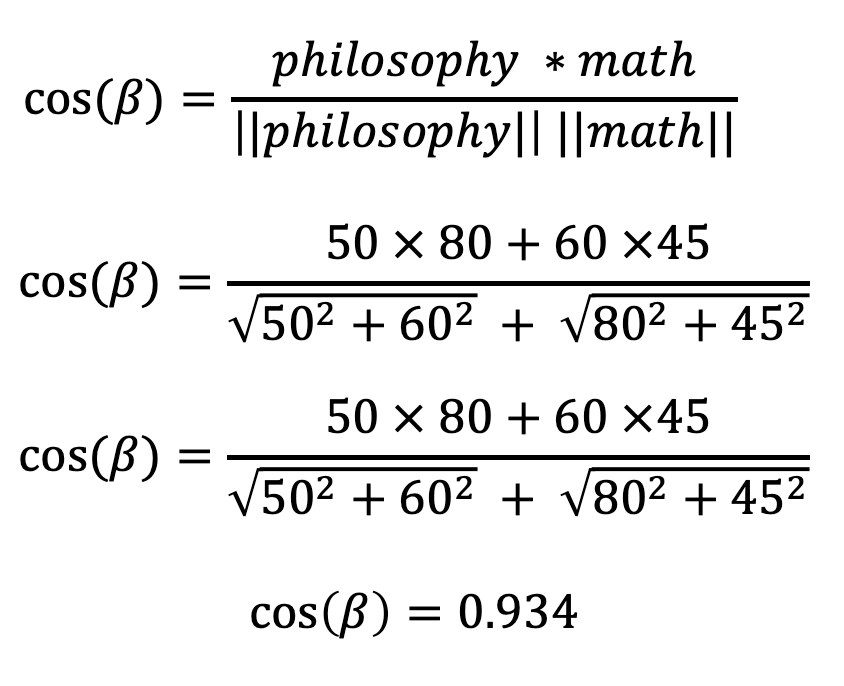Whereas cos(alpha) = 0.99 which is higher than cos(beta) meaning philosophy is closer to psychology than it is to math. 而cos(alpha) = 0.99 (高于cos(beta)意味着哲学比数学更接近心理学。
Recall that 回想起那个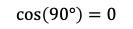and 和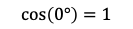This implies that the smaller the angle, the greater your cosine similarity will be and the greater your cosine similarity, the more similar your vectors are. 这意味着角度越小，您的余弦相似度就越大，并且您的余弦相似度越大，向量就越相似。
Python implementation Python实现
import numpy as npmath = np.array([80, 45])philosophy = np.array([50, 60])psychology = np.array([15, 20])cos_beta = np.dot(philosophy, math) / (np.linalg.norm(philosophy) * np.linalg.norm(math))print(cos_beta) 带走 (Takeaway)
I bet you should know by now how Euclidean distance and cosine similarity works. The former considers the straight line distance between two points whereas the latter cares about the angle between the two vectors in question. 我敢打赌，您现在应该知道欧几里得距离和余弦相似度是如何工作的。 前者考虑了两个点之间的直线距离，而后者则考虑了所讨论的两个向量之间的角度。
Euclidean distance is more straightforward and is guaranteed to work whenever your features distribution is balanced. But most of the time, we deal with unbalanced data. In such cases, it’s better to use cosine similarity. 欧几里得距离更简单明了，并且可以保证只要要素分布平衡就可以使用。 但是大多数时候，我们处理不平衡的数据。 在这种情况下，最好使用余弦相似度。
翻译自: https://medium.com/swlh/euclidean-distance-and-cosine-similarity-which-one-to-use-and-when-28c97a18fe68余弦相似度和欧氏距离
展开全文python
• 欧氏距离和余弦距离 参考：https://blog.csdn.net/mr_evanchen/article/details/77511312 一、欧几里得距离(Euclidean Distance) 　欧氏距离是最常见的距离度量，衡量的是多维空间中各个点之间的绝对距离。公式如下...
• 欧氏距离和马氏距离简介 By:Yang Liu 1.欧氏距离 在数学中，欧几里得距离或欧几里得度量是欧几里得空间中两点间“普通”（即直线）距离。欧几里得度量（euclidean metric）（也称欧氏距离）是一个通常采用的距离定义...机器学习 数据分析 matlab
• 文章目录余弦距离与欧氏距离 余弦距离与欧氏距离 在机器学习领域中，通常将特征表示为向量的形式，所以在分析两个特征向量之间的相似性时，常用余弦相似度表示。例如将两篇文章向量化，余弦距离可以避免因为文章的...
• amp;wfr=spider&...欧氏距离是最容易直观理解的距离度量方法，我们小学、初中和高中接触到的两个点在空间中的距离一般都是指欧氏距离欧氏距离 二维平面上点a(x1,y1)与b(x2,y2)间的欧氏距离:...机器学习
• 多标签各向异性3D欧氏距离变换（MLAEDT-3D） 计算一次包含多个标签的1d，2d或3d标签图像的欧几里德距离变换，并支持各向异性尺寸。 好奇输出是什么样子？ 下载！ import edt import numpy as np # e.g. 6nm x 6nm ...
• ## 欧氏距离分类器

千次阅读 2020-01-20 16:25:02
欧氏距离分类器的Matlab实现 欧氏距离分类器是贝叶斯分类器的退化版本，在样本满足一定条件下成立。 代码如下: function cls = mahalanobisClassifier(varargin) [x,m,sigma] = parseInputs(varargin{:}); %...matlab 机器学习
• 欧氏距离里的距离计算： 曼哈顿距离（出租车距离，因为曼哈顿是一个四四方方的城市）中的距离计算： 图1中红线代表曼哈顿距离， 而蓝色和黄色代表等价的曼哈顿距离。 绿色代表欧氏距离，也就是直线距离， 曼哈顿...
• 在特征值比对的时候，会遇到求两个特征向量之间相似度的情况，以下代码为求两个特征向量的欧氏距离然后转换为相似度的几行代码。 1.由于相似度一般是在0-1之间，而欧氏距离可能会很大，所以首先将特征向量归一化到0...
• Mahalanobis Distance & Euclidean Distance Definition   马氏距离（Mahalanobis Distance）是由印度统计学家...与欧氏距离不同的是它考虑到各种特性之间的联系（例如：一条关于身高的信息会带来一条关
• 通常常用的两种度量方式为欧氏距离与余弦距离，那么在什么时候用欧氏距离？什么时候用余弦相似度？他们之间的联系与区别在哪里呢？ 探索 在机器学习当中，通常以一组向量来表示样本 如上图所示，欧式距离是通过...
• 欧氏距离定义： 欧氏距离（ Euclidean distance）是一个通常采用的距离定义，它是在m维空间中两个点之间的真实距离。 在二维和三维空间中的欧式距离的就是两点之间的距离，二维的公式是  d = sqrt((x1-x2)^+(y1...
• 平面上的两个点（1,1）和（3,3），其曼哈顿距离为4，欧氏距离为 曼哈顿距离：在平面中，有x、y两个坐标轴，那么两个点之间的曼哈顿距离，为他们分别投影到两个坐标轴上的距离的绝对值之和，坐标轴更多则加数更多。 ...曼哈顿距离 室内定位
• % 计算样本点云中每个点的欧氏距离为% 参考点云中最近的点（列数必须％ 比赛） ％ 输入： % 参考 = M x N 矩阵% 样本 = P x N 矩阵 ％ 输出： % distMat = P x N 矩阵matlab
• 对于两个向量A和B，其余弦相似度...当一对文本相似度的长度差距很大、但内容相近时，如果使用词频或词向量作为特征，它们在特征空间中的的欧氏距离通常很大；而如果使用余弦相似度的话，它们之间的夹角可能很小，因...机器学习 人工智能 python 深度学习 java
• 欧氏距离通常携带有量纲，因此量纲的影响会对距离的计算结果产生很大影响。 比如如果携带单位的话，两对坐标点是 A(0,5),B(10,0) C(0,10),D(1,0) 如果同度量的话，比如都是cm，AB距离为,CD之间的距离为,AB的距离...
• mport math len = math.hypot(x1-x2, y1-y2) #（x1, y1）与（x2, y2）间的欧氏距离
• Atitti knn实现的具体四个距离算法 欧氏距离 余弦距离 汉明距离 曼哈顿距离
• 1. 欧氏距离 绝对距离。 2. 余弦距离 角度。 归一化后的欧式距离和余弦距离关系： 参考： 欧氏距离和余弦相似度的区别是什么？
• 首先给出结论，归一化后的向量的余弦距离和欧氏距离是等价的。 公式敲起来很麻烦，这里我就不敲了，引用一位知乎答友的图。链接在这了欧氏距离和余弦相似度的区别是什么？ 上面的公式可以再推一步： euc=2(1−cos(A......